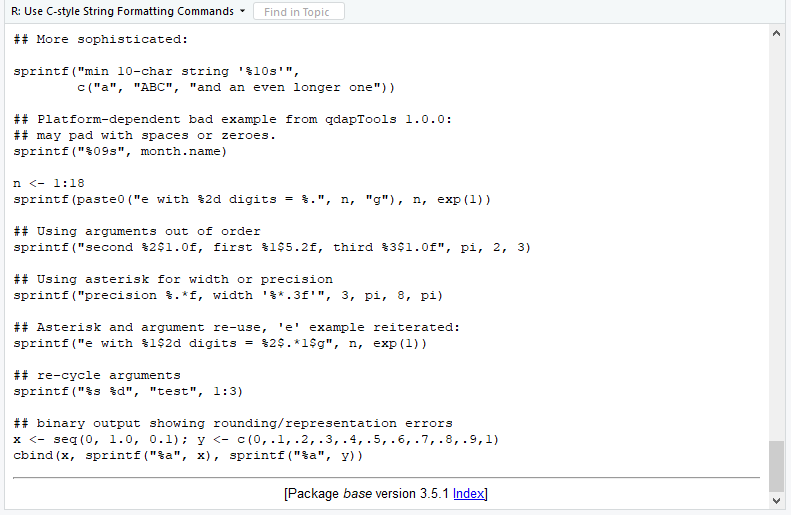# sprintf R Function (6 Example Codes)

This article explains how to apply the sprintf() function in R. First, let’s have a look at the basic R syntax and the definition of sprintf:

Basic R Syntax of sprintf:

`sprintf("%f", x)`

Definition of sprintf:

The sprintf function returns character objects containing a formatted combination of input values.

In this tutorial, I’ll show you based on six examples how to use sprintf in the R programming language.

Let’s move straight to the examples…

## Example 1: Format Decimal Places with sprintf Function in R

The first example explains how to modify the number of decimal places with sprintf. I’m going to use the following numeric data object for the examples of this tutorial:

`x <- 123.456               # Create example data`

We can now use sprintf to format the decimal places. The default number of decimal places is printed as follows (i.e. six digits after the decimal point):

```sprintf("%f", x)           # sprintf with default specification
#  "123.456000"```

We can control the number of decimal places by adding a point and a number between the percentage sign and the f. For instance, we can print ten digits after the decimal point…

```sprintf("%.10f", x)        # sprintf with ten decimal places
# "123.4560000000"```

…or we can round our numeric input value to only two digits after the decimal point:

```sprintf("%.2f", x)         # sprintf with two rounded decimal places
# "123.46"```

Note: The output of sprintf is a character string and not a numeric value as the input was.

## Example 2: Format Places Before Decimal Point

sprintf also enables the formatting of the number of digits before the decimal separator. We can tell sprintf to print all digits before the decimal point, but no digits after the decimal point…

```sprintf("%1.0f", x)        # sprintf without decimal places
# "123"```

…or we can print a certain amount of leading blanks before our number without decimal places (as illustrated by the quotes below)…

```sprintf("%10.0f", x)       # sprintf with space before number
# "       123"```

…or with decimal places…

```sprintf("%10.1f", x)       # Space before number & decimal places
# "     123.5"```

…or we can print blanks at the right side of our output by writing a minus sign in front of the number within the sprintf function:

```sprintf("%-15f", x)        # Space on right side
# "123.456000     "```

## Example 3: Print Non-Numeric Values with sprintf (e.g. + or %)

It is also possible to combine numeric with non-numeric inputs. The following R code returns a plus sign in front of our example number…

```sprintf("%+f", x)          # Print plus sign before number
# "+123.456000"```

…and the following R code prints a percentage sign at the end of our number:

```paste0(sprintf("%f", x),   # Print %-sign at the end of number
"%")
# "123.456000%"```

## Example 4: Control Scientific Notation

The sprintf R function is also used to control exponential notation in R. The following syntax returns our number as scientific notation with a lower case e…

```sprintf("%e", x)           # Exponential notation
# "1.234560e+02"```

…and the following code returns an upper case E to the RStudio console:

```sprintf("%E", x)           # Exponential with upper case E
# "1.234560e+02"```

## Example 5: Control Amount of Decimal Zeros

We can also control the amount of decimal zeros that we want to print to the RStudio console. The following R code prints our example number without any decimal zeros…

```sprintf("%g", x)           # sprintf without decimal zeros
# "123.456"```

…the following R code returns our example number * 1e10 in scientific notation…

```sprintf("%g", 1e10 * x)    # Scientific notation
# "1.23456e+12"```

…and by adding a number before the g within the sprintf function we can control the amount of decimal zeros that we want to print:

```sprintf("%.13g", 1e10 * x) # Fixed decimal zeros
# "1234560000000"```

## Example 6: Several Input Values for sprintf Function

So far, we have only used a single numeric value (i.e. our example data object x) as input for sprintf. However, the sprintf command allows as many input values as we want.

Furthermore, we can print these input values within more complex character strings. Have a look at the following sprintf example:

```sprintf("Let's create %1.0f more complex example %1.0f you.", 1, 4)
# "Let's create 1 more complex example 4 you."```

The first specification (i.e. %1.0f) within the previous R code corresponds to the input value 1 and the second specification corresponds to the input value 4.

Of cause we could use sprintf in even more complex settings. Have a look at the sprintf examples of the R help documentation, if you are interested in more complex examples:Figure 1: Complex sprintf Examples in R Help Documentation.

## Tutorial Video & Further Resources for Dealing with Character Strings

If you still have questions concerning the method I used in this tutorial, feel free to watch the video on my YouTube channel, where I describe the syntax more detailed:

Please accept YouTube cookies to play this video. By accepting you will be accessing content from YouTube, a service provided by an external third party.If you accept this notice, your choice will be saved and the page will refresh.

The sprintf function is obviously only one of many R functions for the Handling of character strings. A similar function to sprintf is the paste function. If you want to learn more about paste, you could have a look at the following YouTube video of Jonatan Lindh:

Please accept YouTube cookies to play this video. By accepting you will be accessing content from YouTube, a service provided by an external third party.If you accept this notice, your choice will be saved and the page will refresh.

Also have a look at the other tutorials of this website. I have already published several tutorials on the handling of character strings in R:

This tutorial showed you how to use the sprintf function in R. In case you have any further questions, let me know in the comments below.

Subscribe to the Statistics Globe Newsletter